# Machine learning as a tool for evidence-based policy

## By Dan Steinberg & Finn Lattimore

In this post we discuss how in certain situations machine learning can be a useful tool for observational causal inference studies, one of the cornerstones of evidence-based policy. Firstly we introduce what an observational study is and how we can estimate causal effects from observational data using a running example. We then discuss how machine learning can help us capture complex relationships in the data, thereby helping mitigate bias from model mis-specification. Finally we show that a trick commonly used in machine learning, regularisation, can lead to causal estimates with less error compared to unbiased methods when we have many related confounding factors in our data.

Experimentation is fundamental to learning and to progress. Babies and animals experiment through play in order to learn how to move, interact with others and ultimately survive. It is hard to dispute that experimentation is an effective tool for inferring cause and effect, and yet many policies created by organisations and governments are not informed by experimentation. This may sometimes be for ideological and political reasons, but it may also be that performing experiments in certain policy settings can be prohibitively expensive, complex, unethical or simply impossible. For example, policy on the regulation of smoking and leaded petrol was largely not informed by experimental data, since exposing people to these substances was already suspected to cause harm. In these situations we must either identify some external factor that creates a natural experiment, or resort to using data that has been collected in the absence of any experimental intervention — such data is known as observational data.

When we analyse observational data, all we can measure are correlations, or factors that vary in similar ways to each other within the data. Say we are analysing a dataset from an education system and in it are factors relating to the experience of teachers in the school system, demographic backgrounds of students, and test scores. We would like to determine how much teacher experience affects test scores, since this could influence future policy on how teachers are assigned to schools. When we analyse this dataset we see that less experienced teachers tend to be associated with students who have lower test scores. This may lead us to conclude that teachers with less experience cause students to have lower test scores. If we look further we may also find that there is a correlation between demographic background and test scores — disadvantaged students tend to have lower scores — and there is a correlation between disadvantaged students and teachers with less experience. Now we don’t know whether teacher experience is causing low test scores, or if demographic disadvantage is causing low test scores and also somehow affecting teacher experience. This data can’t tell us what is causing what. If we could intervene and randomly assign high and low experience teachers to students we would break the link with demographics, but such an experiment sounds expensive and fairly controversial! So we are left in a situation where we have data that is confounded; where there are common causes for factors we want to measure, and we cannot readily separate these causes from one another.

All is not lost however! Econometricians and statisticians have been studying confounded data for decades, and have devised methods in which causal effects from such data may be inferred. The foundation for these methods is the idea that we, the data scientists and domain experts, can reason about the direction of the causal relationships in the data. That is to say, we have theories about the relevant causal relationships. Continuing the example above, say we interview a teacher about the education system and they tell us that junior teachers have less choice about where they work and are more likely to be employed in schools in disadvantaged areas. Once they gain experience they tend to move to more advantaged schools. Education research also tells us that a parent’s level of education has an influence both on the family’s social advantage and their childrens’ academic performance. This now gives us a clue as to the causal relationships in our data — demographic background influences both test scores and which schools teachers are employed. Figure 1 illustrates this theorised relationship.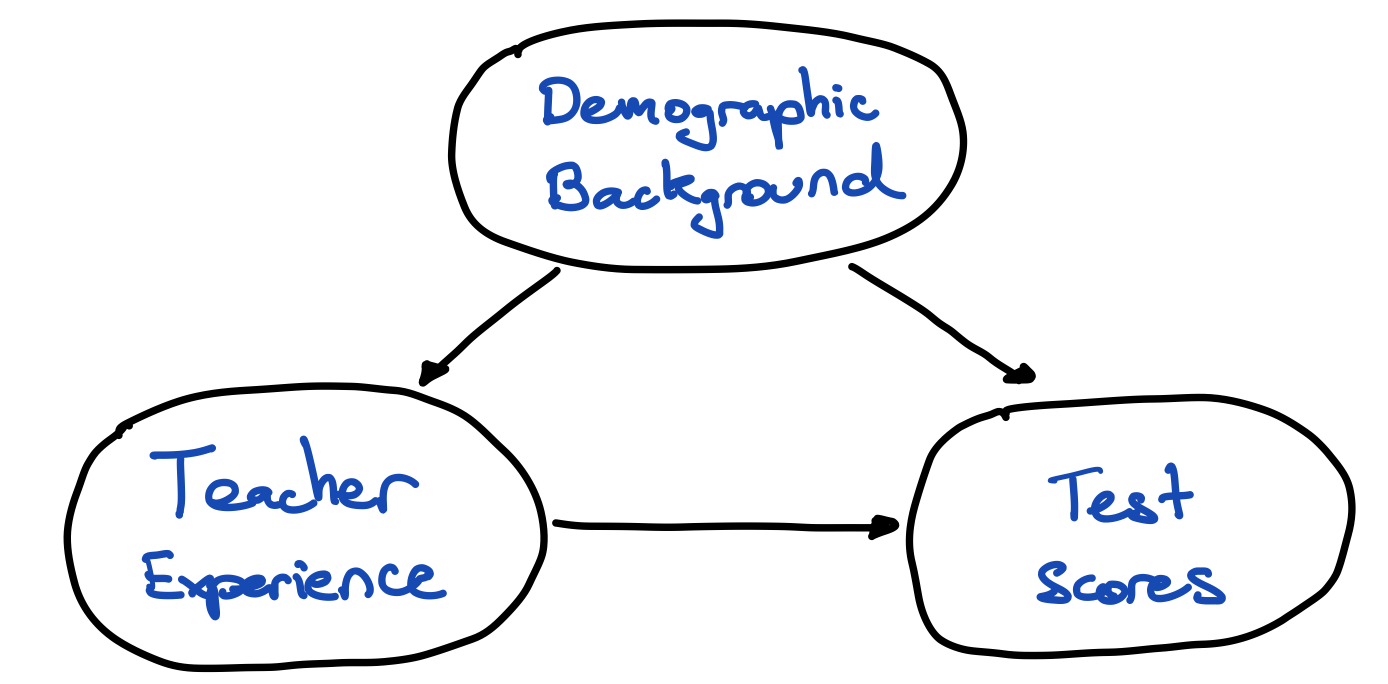Figure 1 — theorised causal relationships in an example education system. Student demographic background is a common-cause of students’ performance, and which schools teachers are employed.

The aforementioned methods for observational causal inference tell us that if we can identify all common causes, also called confounding factors, we can control for them [TN1, TN2]. This means that if we can measure all common causes we can remove their influence on the relationship between the treatment (teacher experience) and the outcome (test scores)!

There are many methods used for controlling these confounding factors, and one of the most common and simplest to apply is regression. We’re going to introduce some notation now, which will hopefully help us clarify some concepts later. Let’s call our treatment variable (teacher experience) Z, the outcome of interest (test score) Y, and the confounding factor (demographic background) X. Our theoretical causal model may then be;

Y = αZ + βX + ε

In words: an individual’s outcome, Y, is the result of α-units of the treatment, Z, plus β-units of the confounder, X, plus some unrelated random noise ε. Now we can see that the causal effect of the treatment on the outcome is α. That is, if we change Z by one unit, we change Y by α units. As long as we include all common causes of Y and Z into this model as X, we can use regression to estimate the causal effect α and the relationship β [TN3]. We have depicted this relationship in Figure 2. Another caveat of this method is that we have to specify the theoretical causal model correctly [TN4] — this means that if, in this instance, Y is not a weighted sum of Z and X then our estimate of the causal effect, α, will be wrong — more on this later.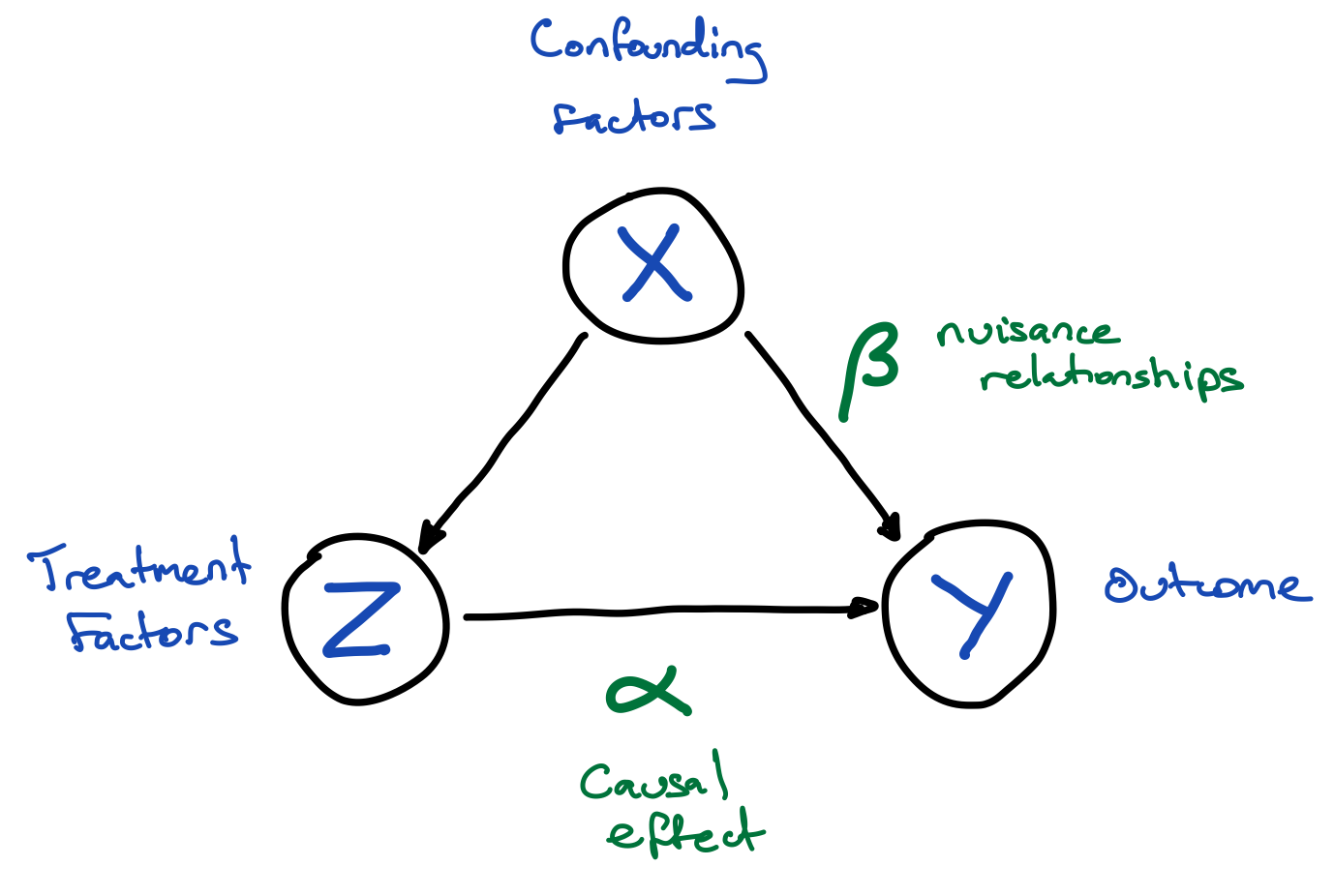Figure 2 — Graphical representation of the relationships assumed in the theoretical causal model. We estimate α and β using regression, α is the causal effect of the treatment (Z) on the outcome (Y) and the quantity we are interested in. β are a nuisance because we have to estimate them to control for the confounding factors (X), but strictly speaking we don’t care about their values since they are not directly relevant to the question we are asking: “how does the treatment affect the outcome”.

So now we have presented a method for inferring causal effects from observational data, but we have also mentioned two caveats when we use this method,

1. Our theoretical causal model that specifies how all of the factors in our data relate to one another has to reflect the real-world causal relationships.
2. We have to measure and model all confounding factors (common causes of the treatment and outcome we are interested in).

Failing to make a theoretical causal model reflect reality leads to what is known as model mis-specification bias. Failing to measure and model all confounding factors leads to confounding bias. Machine learning algorithms can actually help to avoid issues associated with both of these problems. We will now delve into how they do this.

# Machine learning can help with complexity

Classical techniques for regression are typically quite limited in the sorts of relationships they can capture. “Out of the box” most of these techniques are limited to modelling linear relationships between the inputs (controls X, treatments Z) and the output (Y) — for example the plot on the left in Figure 3, and the additive model we introduced previously.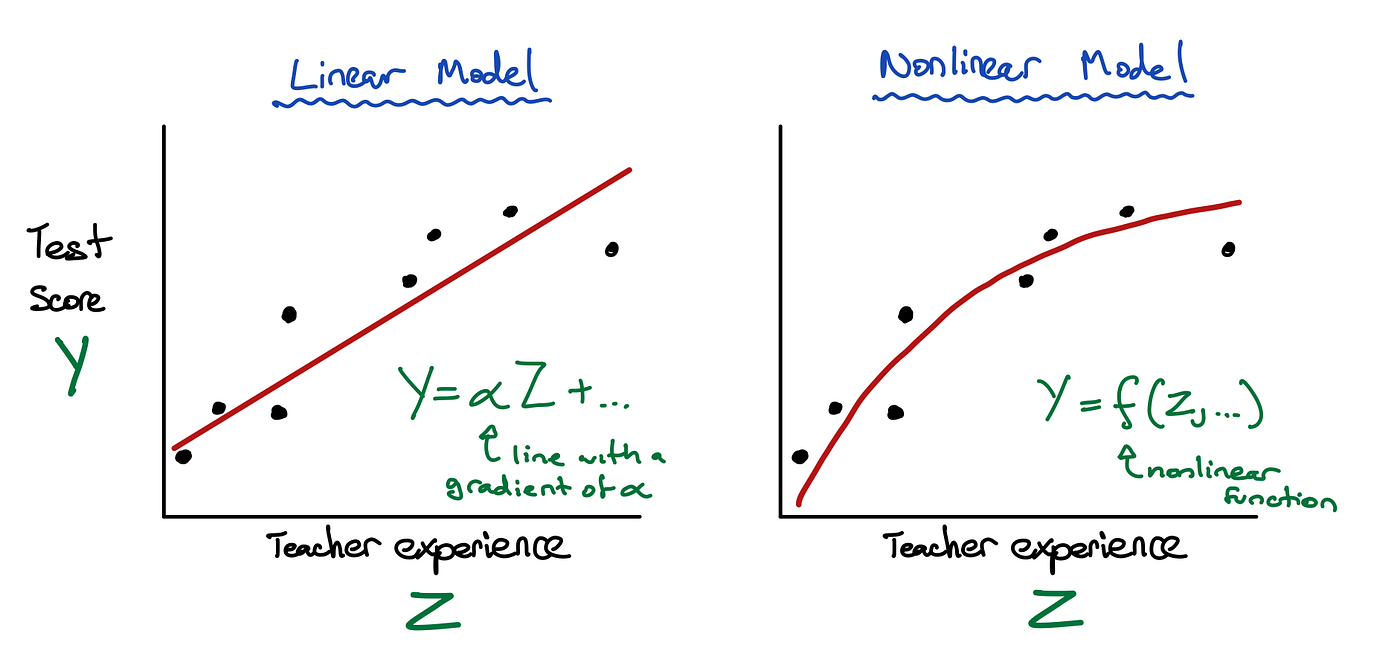Figure 3 — Comparing how linear (left) and nonlinear (right) models fit the same set of individuals’ data. The points are the individual data, the curves are the curves of best fit from each model. The linear model is limited to relationships that have a constant rate of increase/decrease. In this case if we were to increase the experience of the teacher we would expect to see a constant α-units of change in their student’s score, where α is the slope of the line. However, if we look at the nonlinear model on the right, we can see now there is a diminishing relationship between teacher experience and a student’s score. In other words, the rate of improvement in student test scores is greatest for inexperienced teachers, and less for experienced teachers. Typically nonlinear relationships cannot be explained with one number, unlike a linear relationship. These models suggest quite different policies for school teacher assignment. The nonlinear model suggests that more overall improvement could be made to student test scores by increasing the level of experience of the teachers at schools with a greater mix of inexperienced teachers. In both cases we are controlling for confounding factors (X).

We can adapt these classical techniques to fit nonlinear relationships (e.g. the right plot in Figure 3), but we have to explicitly choose the sorts of nonlinear relationships we want to fit and then apply them manually to the inputs of a model. This increases the burden on the data scientist, especially if there are many confounding factors to control for, and where each factor can interact nonlinearly with any other factor. The complexity of the modelling task increases exponentially with the number of factors we need to control for. If we get these relationships wrong, we will bias our results.

Machine learning research has been tackling this specific nonlinear modelling problem for decades. There is literally a plethora of machine learning algorithms that can search for “patterns” (nonlinear relationships) in high-dimensional data (data with a lot of factors). We won’t go into details here, but some well known examples of these algorithms are additive decision trees, kernel machines, and neural networks. Machine learning models provide an alternative, more flexible approach to causal inference. One in which data scientists can let machine learning algorithms learn the complex nuisance relationships between the confounding factors, freeing them to concentrate on modelling the treatment/outcome relationship (if they wish, they can also use machine learning for it).

# Machine learning can help with control

Using machine learning to help model the nuisance relationships between many confounding factors is not the only way it can help with causal inference. As a society we are collecting more data on people and the natural world than ever before. Consequently we have available to us more data for use as controls in observational causal studies. In the running example we have identified one confounding factor; student demographic background. Realistically this concept would be captured from a number of factors such as the parent’s occupations and educational attainment, household income and health indicators. Also teacher experience and student test scores would probably be confounded by a number of other factors, such as if a school was selective, the morale of the staff, and the quality of the school leadership. We can see that to even answer a simple question such as “does teacher experience affect student test performance” requires us to measure tens to hundreds of confounding factors! Machine learning can be used to help deal with the complexity associated with these additional factors. Even if all of these confounding factors, our treatment, and the outcome were linearly related, classical regression techniques may still fail when we must adjust for many confounding factors!!

The reason classical regression techniques can struggle with many confounding factors is fairly nuanced, but we will try to give the reader a feel for the problem, and why machine learning can help. The concept of demographic background from our running example is useful for understanding this issue. It is a relatively simple concept; we may expect people from a disadvantaged background on average to have lower income, less education, and poorer health than those with an advantaged background. It is also a single scale; from low to high. However to “observe” this scale of disadvantage we have to measure multiple factors that covary; such as income, education, health etc. As we measure more factors about someone, we can create the scale of disadvantage more accurately. But also as we measure more factors, we will probably get diminishing returns on how useful each factor is for the scale. This is because there is often some redundancy in the information between factors when they co-vary [TN5]. It is large amounts of this information redundancy that classical regression techniques can struggle with — in these situations the estimates of the model parameters, including the causal effects (e.g. estimates of α and β) can become very sensitive to the input confounding and treatment factors. Why is this sensitivity bad? Well, it means that if our data changes only a little bit, say some people drop out of our data sample and others come in, it can result in a wild change in the estimate of the causal effect. We know that in these situations causal effect estimates from these techniques can have a high amount of error [TN6]. This is not a desirable property since we cannot trust these causal effect estimates enough to base policy decisions on!

So how do we fix this problem? Before machine learning, a common fix was to select only the “most important” factors to use as controls (and there are different definitions of important). One selection criterion is to only use the factors that covary the least, or to put it another way, have the least amount of information redundancy between them. There are a few problems with this approach:

• It is incredibly hard to search through all of the control factors to select those that satisfy this criteria, especially if there are many of them.
• There is a real danger that leaving the covarying factors out of the model will still confound the analysis. Some important confounders may just covary and there isn’t much we can do about it.
• It is possible to make the causal effect estimate overconfident by doing this (by artificially boosting the statistical degrees of freedom [TN7]).

Rather than hand selecting factors to include in our models to fix the estimation stability issue, we can use a trick commonly used in machine learning: regularisation. Regularisation can be understood as a way to limit the complexity of the relationships a machine learning model can learn. It is one mechanism that is used to limit machine learning models overfitting the data they are trained on — that is, fitting noise or learning spurious relationships from redundant information. The stability issue that classical regression techniques suffer from when they use many confounding factors is a symptom of overfitting the data, even though they are relatively simple compared to many machine learning models. When regularisation is used with a linear regression model it has the effect of suppressing how large the model parameters (e.g. estimates of α and β) can get, thereby making them less sensitive to the data they were learned from. Some forms of regularisation (e.g. lasso) even push a subset of these parameters to zero, essentially selecting spurious factors to drop out of the model automatically! So why isn’t regularisation used all the time? Because it has the potential to bias the causal effect estimates (α), and sometimes in counter-intuitive ways. We argue though that when used judiciously and in the correct settings, regularisation can actually result in less overall error in the estimates of the causal effect [TN8].

We can demonstrate this point with a simulation. We generated random data from the same causal model as is depicted in Figure 2, but with a large number of confounding factors. All of the relationships are linear, and the true causal effect, α, is 0.2. We change the total number of confounding factors, X, from 50 to 300 in eight increments. The number of samples (individuals) is 500. The confounding factor relationships (β₁ to β₃₀₀) are held constant (apart from scaling for dimensionality), but we randomly generate the confounding factor samples (X). These samples then determine the treatment factor and outcomes up to some random noise. These confounding factor relationships are specifically designed to have redundant information in them; as more confounders are added, there are diminishing returns on the information in them about the treatment and outcome. We pit four regression models against each other; ordinary least squares (OLS) which is the “classical” regression technique, and three linear regression models that have regularisation; lasso, ridge regression and a two-stage regression model specifically designed to have less bias in the estimated causal effect from regularisation. We measure two things as a function of the number of confounders; (1) prediction error, that is the error the regression model has in predicting the outcomes, Y, that it has not seen, and (2) causal effect error, or the error the model has when estimating the true α. The results are summarised in Figure 4, and more information on the experiment is in [TN9].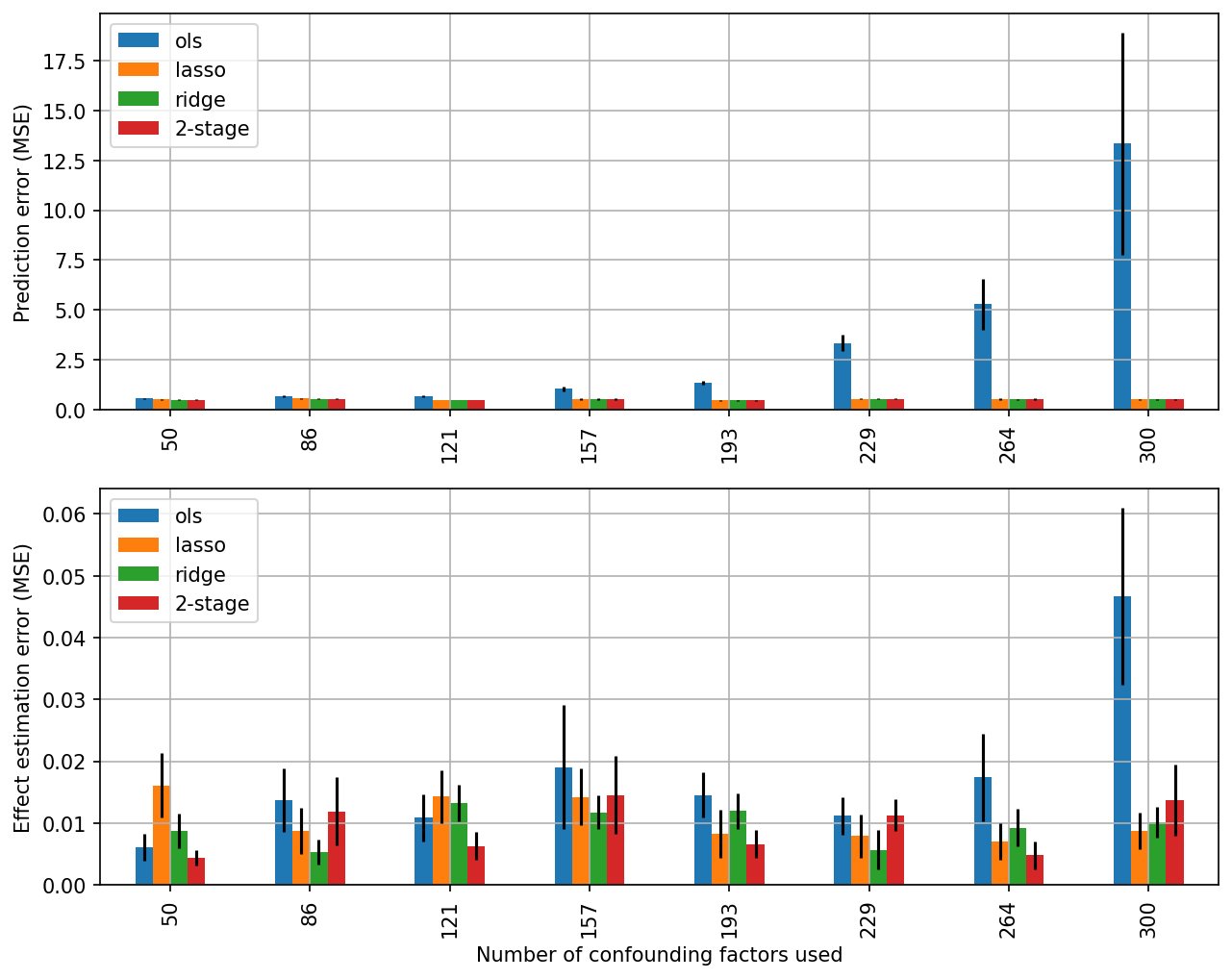Figure 4 — Prediction error (top) and causal effect estimation error (bottom) as a function of the number of confounding factors. OLS is a classical regression method, whereas lasso, ridge and 2-stage all use regularisation to help prevent overfitting. Ten random datasets were generated per number of confounding factors. The black bars are the standard error of the mean-squared error (MSE). The large errors for OLS we see when there are more confounding factors are because of its instability in the face of increased information redundancy.

From the top plot Figure 4 we can see that as we increase the number of confounding factors the prediction error for OLS goes up (gets worse), whereas the regularised regression models continue to perform well. This is because OLS is over-fitting as we give it more redundant information, whereas the regularised model are robust to this issue. The bottom plot in Figure 4 shows the error the models have when estimating the true causal effect and again we can see that OLS tends to have more error with more redundant information than the regularised regression algorithms, even though it is unbiased! What we are seeing here is the error from the instability of OLS estimates when we give it redundant information in the confounding factors [TN8].

# Machine learning is no panacea

In this post we have espoused machine learning as an approach to dealing with issues stemming from the increased availability and complexity of data we are using for observational studies. Machine learning should really be viewed as another tool in the toolbox of inference techniques available to the data scientist. There are still many settings in which classical regression and other techniques are the appropriate tools for performing causal estimation (even if machine learning can do better in prediction). With machine learning’s increased modelling flexibility also comes a decrease in user-friendliness — there are many subtle ways a machine learning algorithm can fail that may be opaque to the uninitiated data scientist. When we use these machine learning tools at Gradient Institute, we typically do so alongside classical methods and with appropriate diagnostics.

When used correctly and carefully however, machine learning can be a powerful tool. It can more reliably capture nuanced and complex relationships that we may not know exist in the data, and may give more reliable estimates of causal effect in the presence of many confounding factors. These attributes can enable the creation of more nuanced and effective policies to bring about change.

# More reading and resources

• Pearl, J., 2009. Causality. Cambridge university press.
• Imbens, G. W. and Rubin, D. B., 2015. Causal Inference for Statistics, Social, and Biomedical Sciences: An Introduction. Cambridge: Cambridge University Press. doi: 10.1017/CBO9781139025751.
• Hill, J.L., 2011. Bayesian Nonparametric Modeling for Causal Inference. Journal of Computational and Graphical Statistics 20, 217–240. https://doi.org/10.1198/jcgs.2010.08162
• Hahn, P.R., Carvalho, C.M., Puelz, D., He, J., 2018. Regularization and Confounding in Linear Regression for Treatment Effect Estimation. Bayesian Anal. 13. https://doi.org/10.1214/16-BA1044
• Chernozhukov, V., Chetverikov, D., Demirer, M., Duflo, E., Hansen, C., Newey, W., Robins, J., 2018. Double/Debiased Machine Learning for Treatment and Structural Parameters. The Econometrics Journal, Volume 21, Issue 1. https://doi.org/10.1111/ectj.12097
• Code for the experiment and the two-stage ridge regression model (Hahn et. al, 2018): https://github.com/gradientinstitute/twostageridge
• EconML — Machine learning algorithms specifically designed for causal inference: https://github.com/microsoft/EconML

# Technical Notes

[TN1] This is known as the ignorability assumption.

[TN2] We actually need one more assumption — common support — which means that there has to be a sufficient level of randomness in our data so that we see similar individuals in all treatment levels of our data. In our example this would mean that we would have to have some disadvantaged students being taught by experienced teachers and vice-versa so we can answer “what-if” style questions still, e.g. “what if all students had inexperienced teachers”.

[TN3] Not just any regression estimator can be used for this. In particular, an unbiased and consistent estimator must be used such as ordinary least squares (OLS), maximum likelihood, generalised method of moments etc. Using such an estimator results in estimated quantities, ᾱ, being unbiased in expectation, E[ᾱ|X, Z] = α. Ridge regression and lasso are not unbiased estimators, though they can be shown to be consistent, that is they converge to the true value α in the large sample limit.

[TN4] Note that we have a continuous treatment variable in our example (e.g. years of teacher experience). If this were a binary or categorical treatment then we could side-step this model mis-specification issue somewhat by using matching, propensity or doubly-robust methods. As far as we are aware, there is not much research on such methods for continuous treatments.

[TN5] More formally, this is multicollinearity. As we use more factors that are linearly related, the condition number of the Gram matrix of the regression inputs WW increases, where W = [Z, X] and W ∈ ℝᴺˣᴰ with N samples, and D dimensions. This makes computing the regression solution for the weights less stable.

[TN6] There is no bias error for an OLS estimator, this error is from the variance of the regression weight estimates, which for OLS is proportional to (WW)ᐨ¹, where W is defined in [TN5]. When the Gram matrix WW has a high condition number [TN5] from multiple related factors we can get arbitrarily large elements in its inverse affecting the variance (and stability) of the estimated regression weights. We talk more about this in [TN8].

[TN7] For example, the degrees of freedom for an OLS regressor is DoF = ND where N is the number of samples, and D the number of input (treatment and controls). By removing controls from the model, we lower D, thereby increasing the degrees of freedom. Lowering the degrees of freedom lowers the regression standard error, s² = SSE / DoF where SSE is the regression sum-of-squared errors. This statistic and DoF is used to compute the statistical significance level of the causal effect estimate, so we can boost the significance of the result by lowering D!

[TN8] This is because of the bias-variance trade-off. When we look at the mean-squared error bias-variance decomposition of the linear regression weights, unbiased estimators (such as OLS) have a zero bias error component. Regularised linear models, such as ridge regressors, have a non-zero bias error component because of the regularisation. However, regularisation also tends to reduce the variance error component of these models. As we saw from [TN5] and [TN6] the OLS variance error component, which is proportional to (WW)ᐨ¹, can explode in magnitude when we have many highly related factors (multicollinearity). Adding some regularisation effectively helps to condition this inverse, keeping the estimate of the regression weights stable, but introduces bias. So the error we are seeing in Figure 4 from OLS is from this variance component of its error.

[TN9] For the complete experimental details we refer the interested reader to the notebook implementation. The basic idea of the experiment is to create a scenario where regularisation is important for prediction and regression coefficient estimation. This is achieved by projecting a low dimensional set of confounding factors (generated from a multivariate Normal distribution) to a high dimensional set using a random projection. We found the random Fourier features projection to be excellent for this task, since the resulting set of projected confounding factors is still full rank, allowing OLS to find a unique solution to the regression coefficients. But these projected confounders have a low effective rank. Effective rank is a measure of the amount of information content (spectral entropy) in a matrix. Figure 5 depicts the effective rank of our projected confounding factors, X, concatenated with the treatment factor, Z, into a matrix W as we increase the projection dimensionality. We can see that as the dimensionality increases, the effective rank exhibits a diminishing, sublinear relationship. This can be interpreted as we increase the dimensionality of the projection, we get a diminishing amount of information about the low dimensional confounding factors. A related property of this projection is that as we increase its dimensionality, the resulting condition number of the Gram matrix WW rapidly increases, which is also plotted in Figure 5. As pointed out in [TN5] this makes the OLS solution for the regression weights less stable, resulting in the high variance errors we see in Figure 4. Adding an amount, λ, to the diagonal of the Gram matrix, WW + λI, which is what ridge regression does, improves the condition number of the resulting matrix, allowing for a stable but biased solution to the regression weights.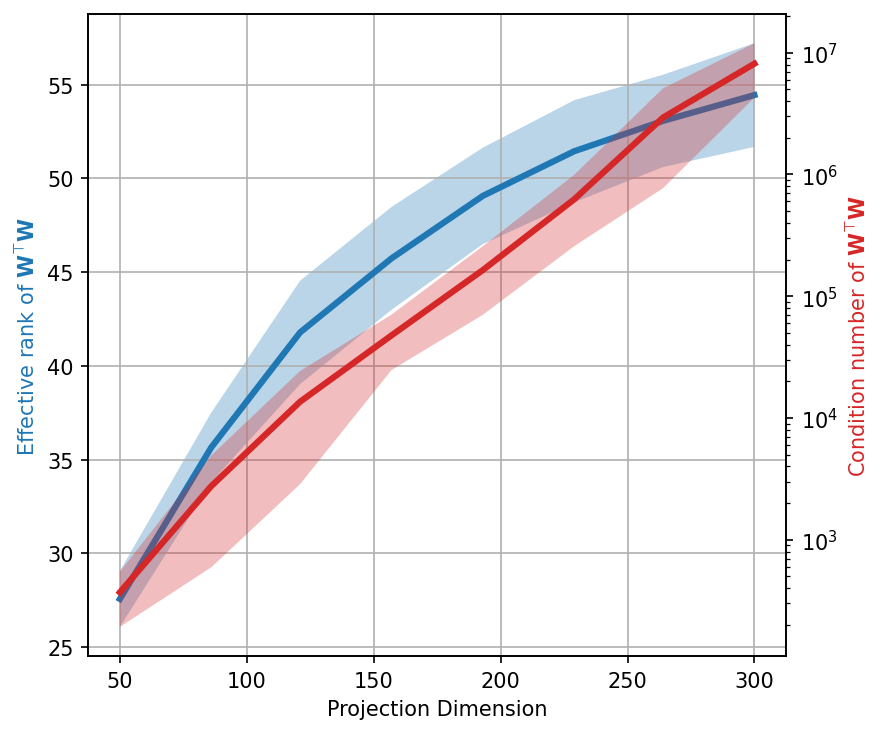Figure 5 — Plot of the effective rank and condition number of the Gram matrix WᵀW as a function of the random Fourier feature projection dimension used in our experiment.

--

--## Dan Steinberg

I am a machine learning researcher at Gradient Institute where I asses, implement and research ethical ML and ML for causal inference.